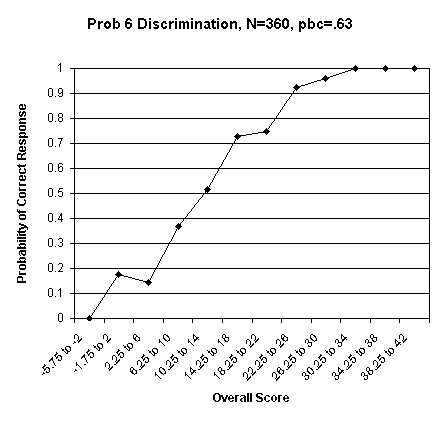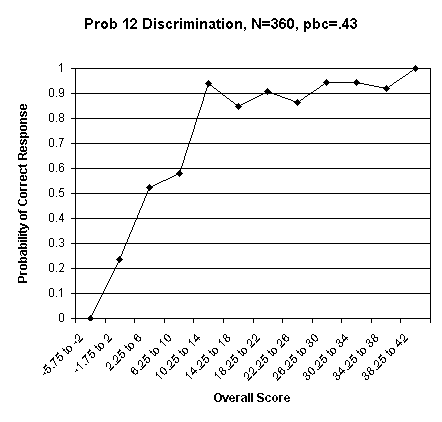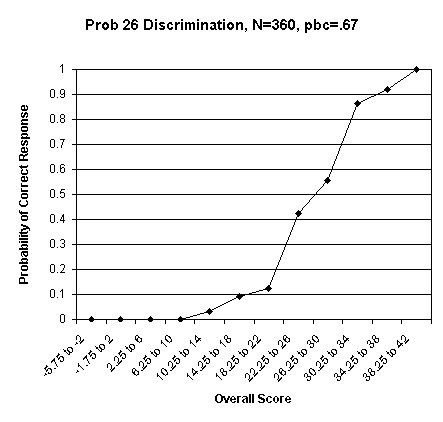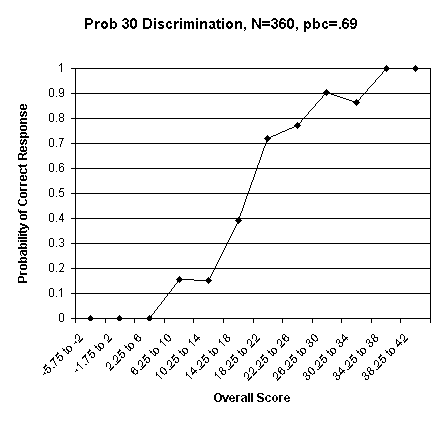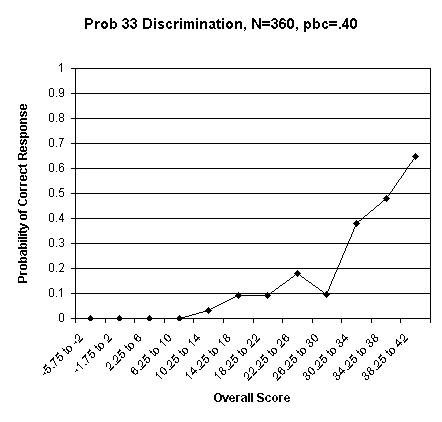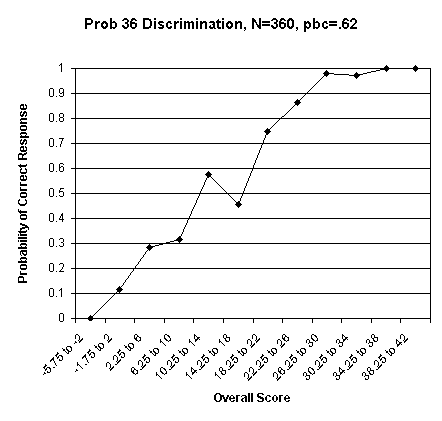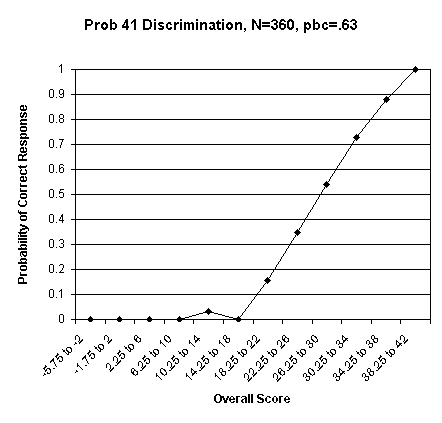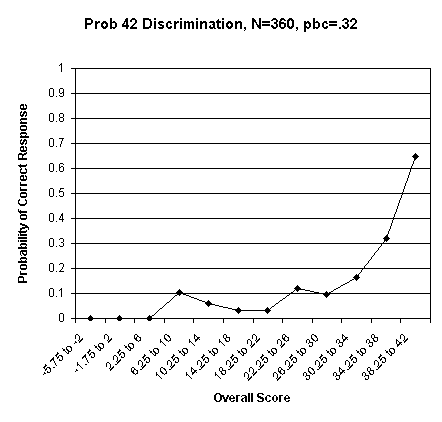## Beta Test Discrimination Plots

pbc = point biserial correlation: the correlation of an individual problem with the total test.
N = number of test-takers in the sample.

These are plots of the number of people getting a particular problem correct (normalized to N) per total-score bin. The bins are distributed as follows:

 Adjusted Score  bin size Number  of scores -5.75 to  -2 5 -1.75 to   2 17 2.25 to   6 21 6.25 to 10 19 10.25 to 14 33 14.25 to 18 33 18.25 to 22 32 22.25 to 26 66 26.25 to 30 52 30.25 to 34 37 34.25 to 38 25 38.25 to 42 20 Total 360